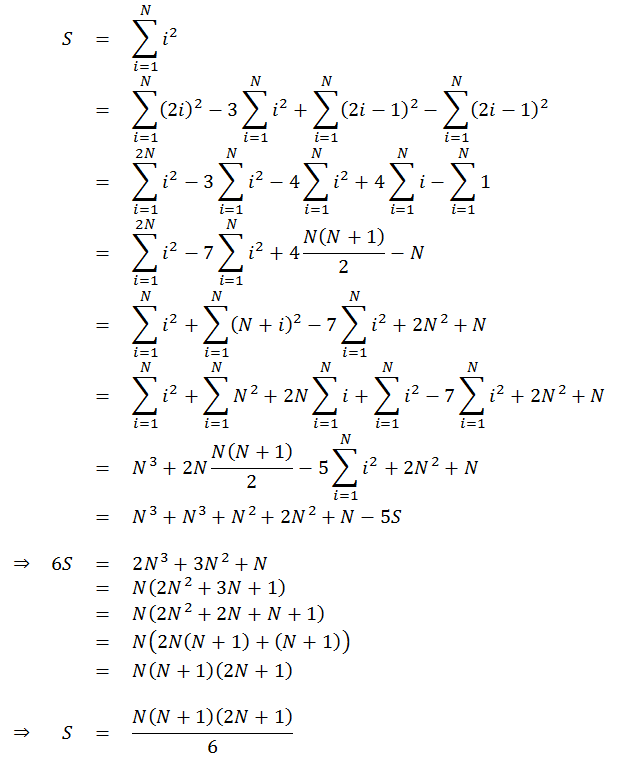# Sum of Squares of First N Numbers

by Zoran Horvat

Given a positive number N, write a function which returns sum of squares of numbers between 1 and N, i.e. 1^2 + 2^2 + ... + N^2.

Example: If N is 5, then return value should be 55 (1 + 4 + 9 + 16 + 25 = 55).

## Problem Analysis

It is easy to produce sum o squares of a sequence by simply iterating through numbers 1 to N. Here is the function which does precisely that:

``````function Sum(n)
begin
sum = 0
for i = 1 to n
sum = sum + i * i
return sum
end
``````

This function runs in O(N) time and O(1) space. In order to improve the running time of the function, we need to find a definite form of the sum of squares. That is possible to do, and here is one of the ways to derive the simple form of the expression:This derivation depends on the equation for calculating simple sum of the numbers 1 to N, which is explained in exercise Sum of First N Numbers . Now the function that runs in O(1) time and O(1) space looks like this:

``````function Sum(n)
begin
return n * (n + 1) * (2 * n + 1) / 6
end
``````

## Implementation

Below is the full listing of a console application in C# which lets the user enter value N and then prints the sum of squares of the sequence.

``````using System;

namespace SumOfSquares
{

public class Program
{

static int Sum(int n)
{
return n * (n + 1) * (2 * n + 1)  6;
}

static void Main(string[] args)
{

while (true)
{

Console.Write("Enter sequence length (zero to exit): ");

if (n <= 0)
break;

Console.WriteLine("Sum of the sequence is {0}\n", Sum(n));

}

}

}
}
``````

## Demonstration

When application is run, it produces output like this:

```            ```
Enter sequence length (zero to exit): 4
Sum of the sequence is 30

Enter sequence length (zero to exit): 5
Sum of the sequence is 55

Enter sequence length (zero to exit): 217
Sum of the sequence is 3429685

Enter sequence length (zero to exit): 0
```
```